# BW-BS balls

Adam has a full box of balls that are large or small, black or white. Ratio of large and small balls is 5:3. Within the large balls the ratio of the black to white is 1:2 and between small balls the ratio of the black to white is 1:8

What is the ratio of all black to all white balls?

x =  0.33

### Step-by-step explanation:

a=1
a+b = 5/3•(c+d)
a = 1/2•b
c = 1/8 • d

a = 1
3a+3b-5c-5d = 0
2a-b = 0
8c-d = 0

a = 1
b = 2
c = 1/5 = 0.2
d = 8/5 = 1.6

Our linear equations calculator calculates it.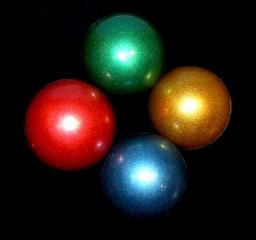Did you find an error or inaccuracy? Feel free to write us. Thank you!Tips to related online calculators
Check out our ratio calculator.
Do you have a system of equations and looking for calculator system of linear equations?

## Related math problems and questions:

• White and black ballsThere are 7 white and 3 black balls in an opaque pocket. The balls are the same size. a) Randomly pull out one ball. What is the probability that it will be white? We pull out one ball, see what color it is and return it to the pocket. Then we pull out th
• Lord Ram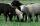When lord Ram founded the breed white sheep was 8 more than black. Currently white sheep are four times higher than at the beginning and black three times more than at the beginning. White sheep is now 42 more than the black. How many white and black shee
• Chess piecesThere are 5 black chess pieces in the box. How many white pieces should we add to this box so that the probability of pulling out a black piece is 1: 4?
• BallsThe urn is 8 white and 6 black balls. We pull 4 randomly balls. What is the probability that among them will be two white?
• Three shapes1/5 of a circle is shaded. The ratio of area if square to the sum of area of rectangle and that of the circle is 1:2. 60% of the square is shaded and 1/3 of the rectangle is shaded. What is the ratio of the area of circle to that of the rectangle?
• BallsPing pong balls have a diameter of approximately 5.1 cm. It sold in boxes of 10 pieces: each box has a cuboid shape with a square base. The balls touch the walls of the box. Calculate what portion of the internal volume of the box is filled with balls.
• Chocolate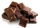I have a box of chocolate - white, milk and dark. The ratio of white to milk with dark is 3: 4. The ratio of white and milk to dark is 17: 4. Calculate what is the ratio between white, milk, dark chocolate.
• EightEight small Christmas balls with a radius of 1 cm have the same volume as one large Christmas ball. What has a bigger surface: eight small balls, or one big ball?
• Alloy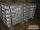The first alloy is a mixture of two metals in the ratio 1:2, the second is a mixture of same metals in the ratio 2:3. At what ratio we have these two alloys put into the furnace to obtain a new metal alloy with ratio 17:27? (All three ratios correspond to
• Holidays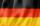Of the 35 students of the class were 7 on holiday in Germany and just as much in Italy. 5 students visited Austria. In none of these countries was 21 students, all three visited by one student. In Italy and Austria were 2 students and in Austria and Germa
• A triangleA triangle has an angle that is 63.1 other 2 are in ratio of 2:5 What are the measurements of the two angles?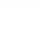When Ben is so many years old to Adam today, Adam will be 23 years old. When Adam was as old as Ben, Ben was two years old. How old is today Ben and Adam?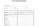At the final exam, the student answers from three areas, which are evaluated in a ratio of 1: 2: 2. What grade will John receive if he answered as follows: 3,1,2.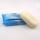Each box has the same number of soaps. A quarter of all boxes contain only white soaps, and in each of the remaining 120 boxes there are always half the white soaps and half the green. White soaps total 1200. (a) the number of all soap boxes; (b) the smal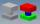In the box are 8 white, 4 blue and 2 red components. What is the probability that we pull one white, one blue and one red component without returning?Grandfather gathered apples into baskets. He filled one large and three small baskets. Small baskets are identical and each can hold 3 kg of apples less than in a large basket. In small baskets together 3 kg of apples over a large basket. How many kilograCha cruz has a garden. The ratio roses to tulips is 2 : 5, the ratio of roses to orchids is 7 : 6. Cha cruz wonders what the ratio of tulips to orchids is. If Cha cruz has 183 plants, how many of each kind are there?